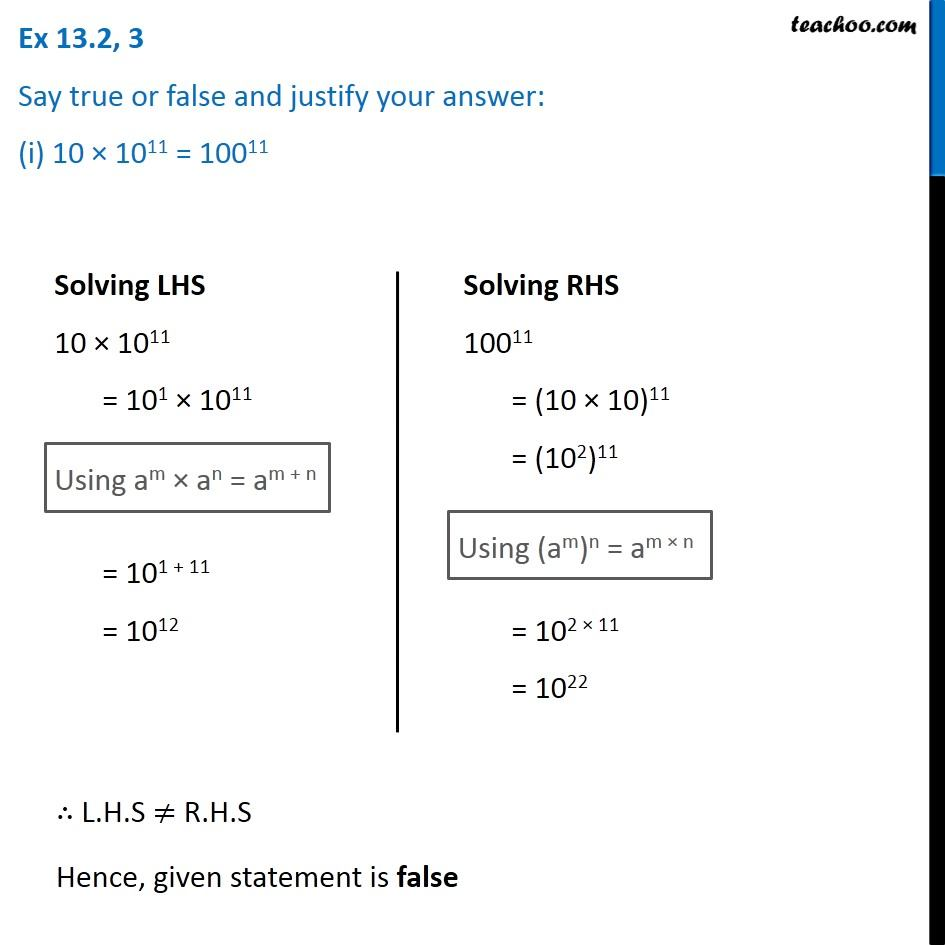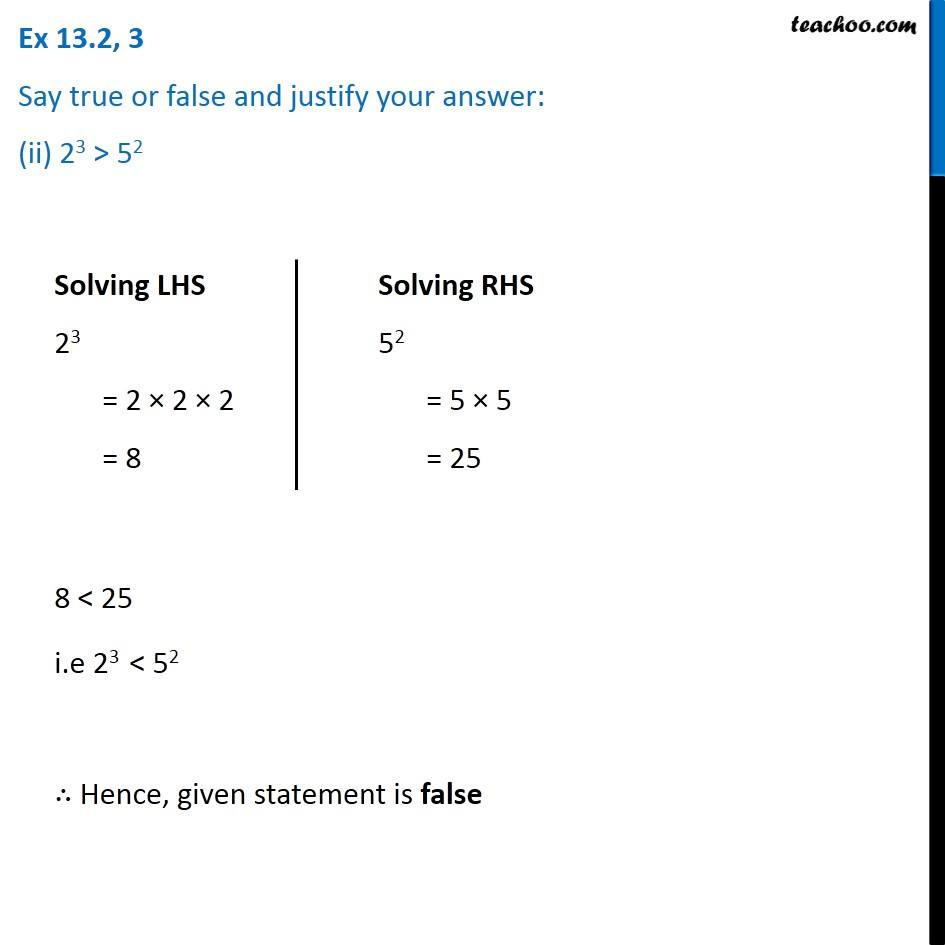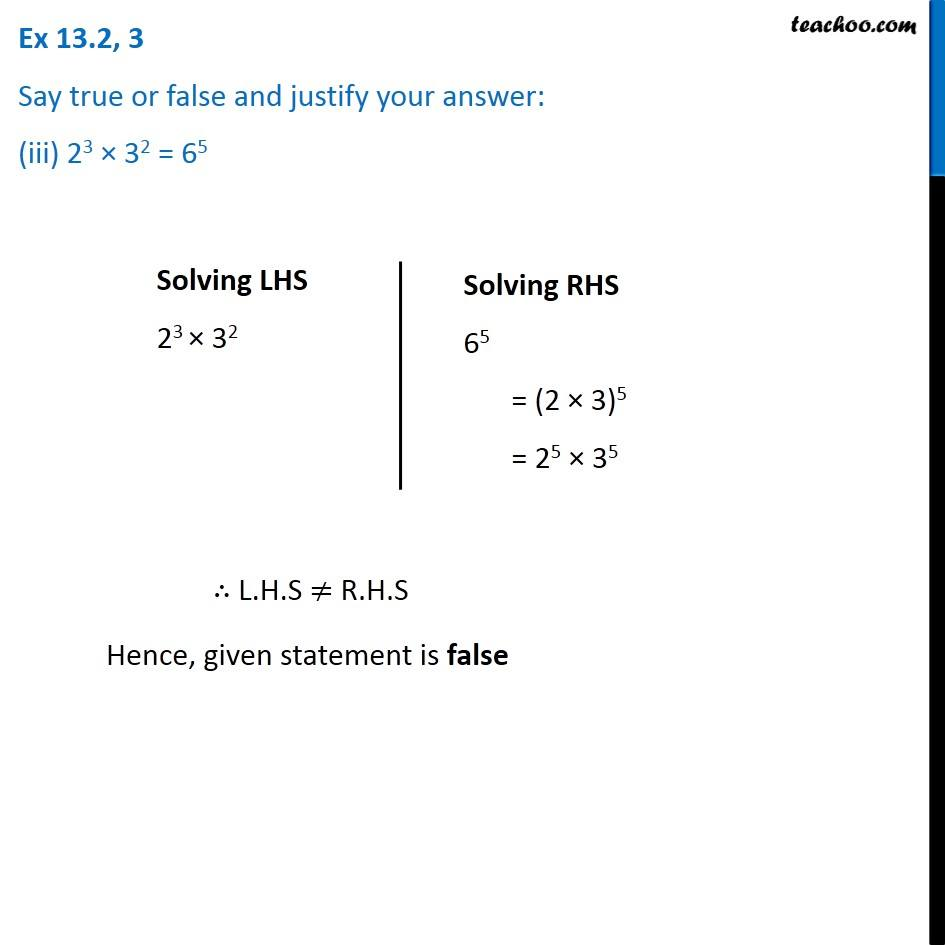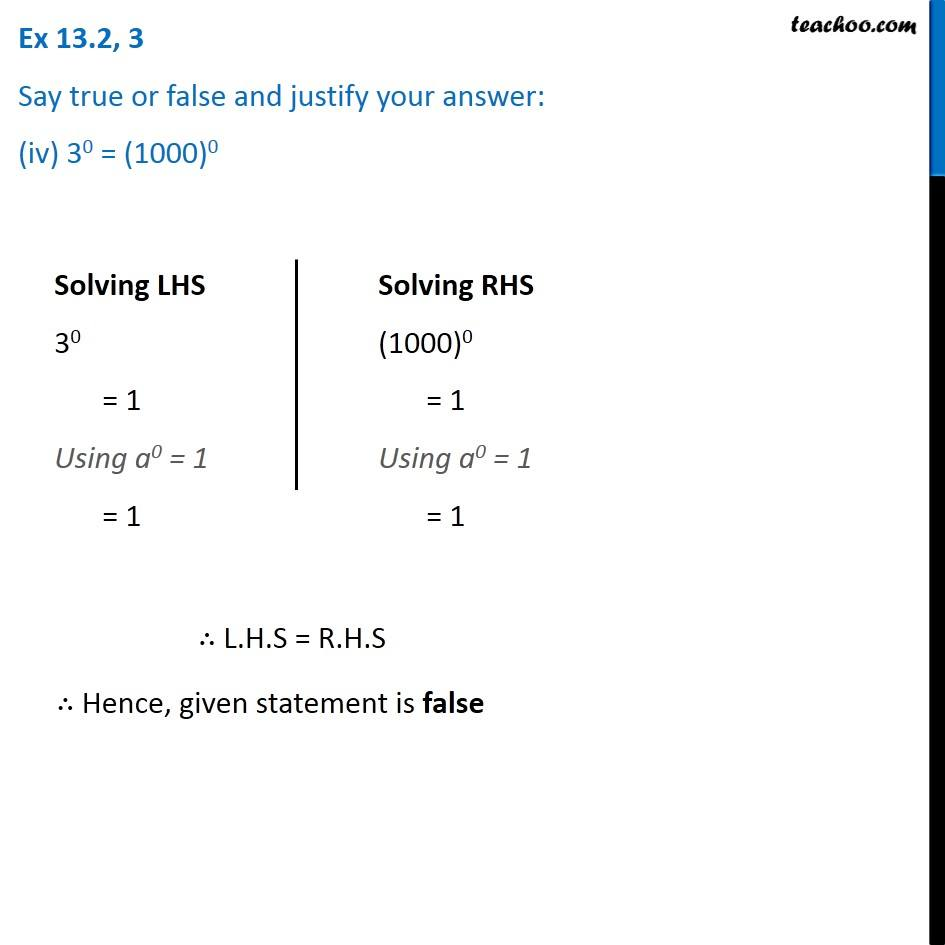1. Chapter 13 Class 7 Exponents and Powers
2. Concept wise
3. Law of exponents

Transcript

Ex 13.2, 3 Say true or false and justify your answer: (i) 10 × 1011 = 10011 Solving LHS 10 × 1011 = 101 × 1011 = 101 + 11 = 1012 Using am × an = am + n = 101 + 11 = 1012 Solving RHS 10011 = (10 × 10)11 = (102)11 Using (am)n = am × n = 102 × 11 = 1022 ∴ L.H.S ≠ R.H.S Hence, given statement is false Ex 13.2, 3 Say true or false and justify your answer: (ii) 23 > 52 Solving LHS 23 = 2 × 2 × 2 = 8 Solving RHS 52 = 5 × 5 = 25 8 < 25 i.e 23 < 52 ∴ Hence, given statement is false Ex 13.2, 3 Say true or false and justify your answer: (iii) 23 × 32 = 65 Solving LHS 23 × 32 Solving RHS 65 = (2 × 3)5 = 25 × 35 ∴ L.H.S ≠ R.H.S Hence, given statement is false Ex 13.2, 3 Say true or false and justify your answer: (iv) 30 = (1000)0 Solving LHS 30 = 1 Using a0 = 1 = 1 Solving RHS (1000)0 = 1 Using a0 = 1 = 1 ∴ L.H.S = R.H.S ∴ Hence, given statement is false

Law of exponents